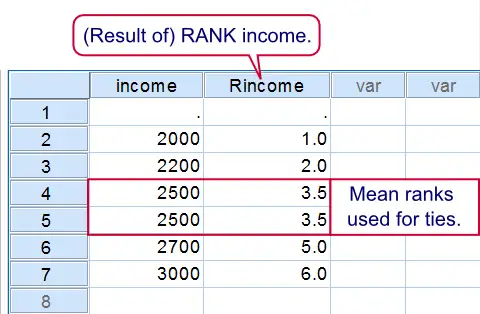SPSS TUTORIALS BASICS ANOVA REGRESSION FACTOR CORRELATION

# SPSS RANK Command

## Summary

SPSS `RANK` can be used to create a variable holding the rank numbers of the values of some other variable. `RANK` is also used for discretizing continuous variables into ntile groups. This tutorial walks you through the main options along with some real world examples.Result of first RANK syntax example

## 1. SPSS Rank Basic Example

Running the syntax below first creates a mini dataset and then ranks `income` (the result is shown in the screenshot). The default name for the new variable is `R` + the old variable name, resulting in `Rincome`. Note that a system missing value does not get any rank number. A value occurring more than once is referred to as a tie. By default, mean rank numbers are assigned to ties.

## SPSS Rank Syntax Example 1

*1. Create mini dataset.

data list free/income.
begin data
3000 2500 '' 2000 2500 2700 2200
end data.

*2. Create variable with rank numbers for income.

rank income.

*3. Sort cases (ascendingly) on income.

sort cases income.

## 2. Creating Ntiles

The following examples will all use employees.sav. The syntax below shows how to create four age groups of equal sizes by using `RANK`. (If the sample size is not divisible by the number of groups or ties are present, SPSS will attempt to make the group sizes as equal as possible.) In first instance the new variable is called `Ndate_of` where the `N` denotes Ntiles.SPSS uses a maximum of 8 letters for new variable names created by `RANK`. This is why we don't get `Ndate_of_birth` (as we may have expected). In step 4, we'll use `MEANS` for inspecting the result.

## SPSS Rank Syntax Example 2

*1. Set default directory and open data.

get file 'employees.sav'.

*2. Create four age groups.

rank date_of_birth
/ntiles(4).

*3. Rename new variable.

rename variables ndate_of = age_group.

*4. Inspect result.

means date_of_birth by age_group
/cells count min max.

## 3. Rank Within Groups

Thus far we used `RANK` with regard to the entire sample. However, we may also `RANK` within groups defined by one or more variables. Like so, the syntax below creates median age groups within each gender separately. When these are combined with `gender` we'll get four groups indicating the 50% youngest women, the 50% oldest women and so on. Note that we undo all changes made to the data thus far by simply reopening it.

## SPSS Rank Syntax Example 3

*1. Reopen data file.

get file 'employees.sav'.

*2. Create median age groups within gender.

rank date_of_birth by gender
/ntiles(2).

*3. Combine age groups with gender.

compute gender_age_group = 2 * gender + ndate_of.

*4. Sorting cases facilitates visual inspection.

sort cases gender date_of_birth.

*5. Apply value labels to new variable.

value labels gender_age_group 1 'Old woman' 2 'Young woman' 3 'Old man' 4 'Young man'.

## 4. Rank Into

In the second example we used a basic `RANK` command and then used `RENAME` for changing the name of the new variable. Alternatively, we can specify a new variable name within `RANK` itself by using the `INTO` keyword as demonstrated by the syntax below.

## SPSS Rank Syntax Example 4

*1. Reopen data file.

get file 'employees.sav'.

*2. Create age_group.

rank date_of_birth
/ntiles(4) into age_group.

## 5. Rank Multiple Variables

If you'd like to `RANK` multiple variables, you can do so in a single command. Like so, the final example below create median groups for `date_of_birth`, `experience_years` and `monthly_income`.

## SPSS Rank Syntax Example 5

*1. Reopen data file.

get file 'employees.sav'.

*2. Create median groups for three variables at once.

rank date_of_birth experience_years monthly_income
/ntiles(2) into dic_age dic_experience dic_income.

## 6. Ranking Descendingly

If you paid close attention, you may have noticed something awkward in the previous example: for `dic_experience` and `dic_income` a higher value indicates more experience/income. However, for `dic_age` the reverse holds. This is because we used `date_of_birth` which is related inversely to age.
One solution is to first compute raw age (also see How to Compute Age in SPSS?) and use that instead. However, an easier way out is to `RANK` (only) date_of_birth descendingly. Simply replacing `RANK date_of_birth` by `RANK date_of_birth (D)` in the last syntax does the trick here.

## Final Note

• Note that ranking into ntiles is quite similar to RECODE. However, with `RANK` the values that separate ntile groups depend on the data whereas with `RECODE` the group sizes depend on the data.

# Tell us what you think!

*Required field. Your comment will show up after approval from a moderator.